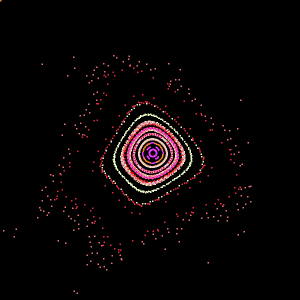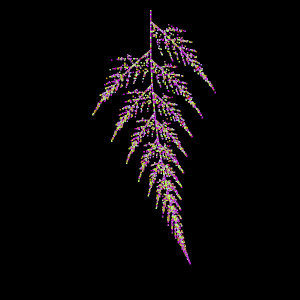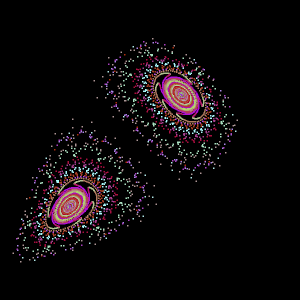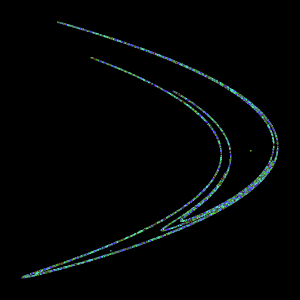Fractal Growth Models

Author: Audrey Karperien (akarpe at sbcglobal.net)
Charles Sturt University, Australia
History: 2005/11/15: First version
Requires: Java 1.3 or later
Source: Fractal_Growth_Models.java
Installation: Download Fractal_Growth_Models.java to the plugins folder, or subfolder, and compile and run it using Plugins/Compile and Run.
Description:

This plugin draws iterated growth models in ImageJ. These are saveable screen drawings made of points calculated using the basic expressions:
xn+1 = mxnXxpow + nynXypow + o
and
yn+1 = fxnYxpow + gynYypow + h.

For each model, each equation's coefficients (m,n,o;f,g,h; Xxpow, Xypow, Yxpow, Yypow) are specified in sets that the user can modify. Each of these sets also contains a probability of using the set. This is for cases when more than one set of coefficients is used to calculate each point, chosen according to a likelihood that the user sets.

Each particle calculated and drawn is coloured on a black background, and all are the same size. Colours are generated randomly, without user input. The user can change the size of the particles in the models, however, using an option in the first popup.

Models

There are five default models. The user can change the coefficients to vary the basic pattern, or change the number of sets to create a new model.

• The "Henon" model is specified by one set of coefficients (i.e., the likelihood of using that set is 1).
• The Henon Map model is also specified by one set of coefficients. It has a (capacity) fractal dimension that is assumed to be around 1.261 (correlation dimension around 1.25).
• The "Random" model is similar to the Henon models, but uses two sets of coefficients and probabilities.
• The "D. Greene Fern" model uses four sets of coefficients. It is from an algorithm by David Greene (Charles Sturt University),
• The custom model is essentially the same as the fern, with a few changes in coefficients and probabilities.

### Using the plugin

1. To generate a default model, the user selects a model from the dropdown menu on the first popup. The number of sets for the selected model is automatically determined, and this number will appear in the next popup.
2. If the user wants to modify a model, the number in this second popup can be changed.
3. After a number of sets is selected, one popup appears for each set. The popups show each coefficient's name listed beside a textbox for its value. The values can be left at the defaults, or changed as desired.
4. At the bottom of each of these popups, there is a space for the probability that the coefficients above it will be used in generating each next point. The total of all probabilities should not be greater than 1; all sets for values that make the cumulative total greater than 1 are ignored.

### Examples|Plugins | Home |